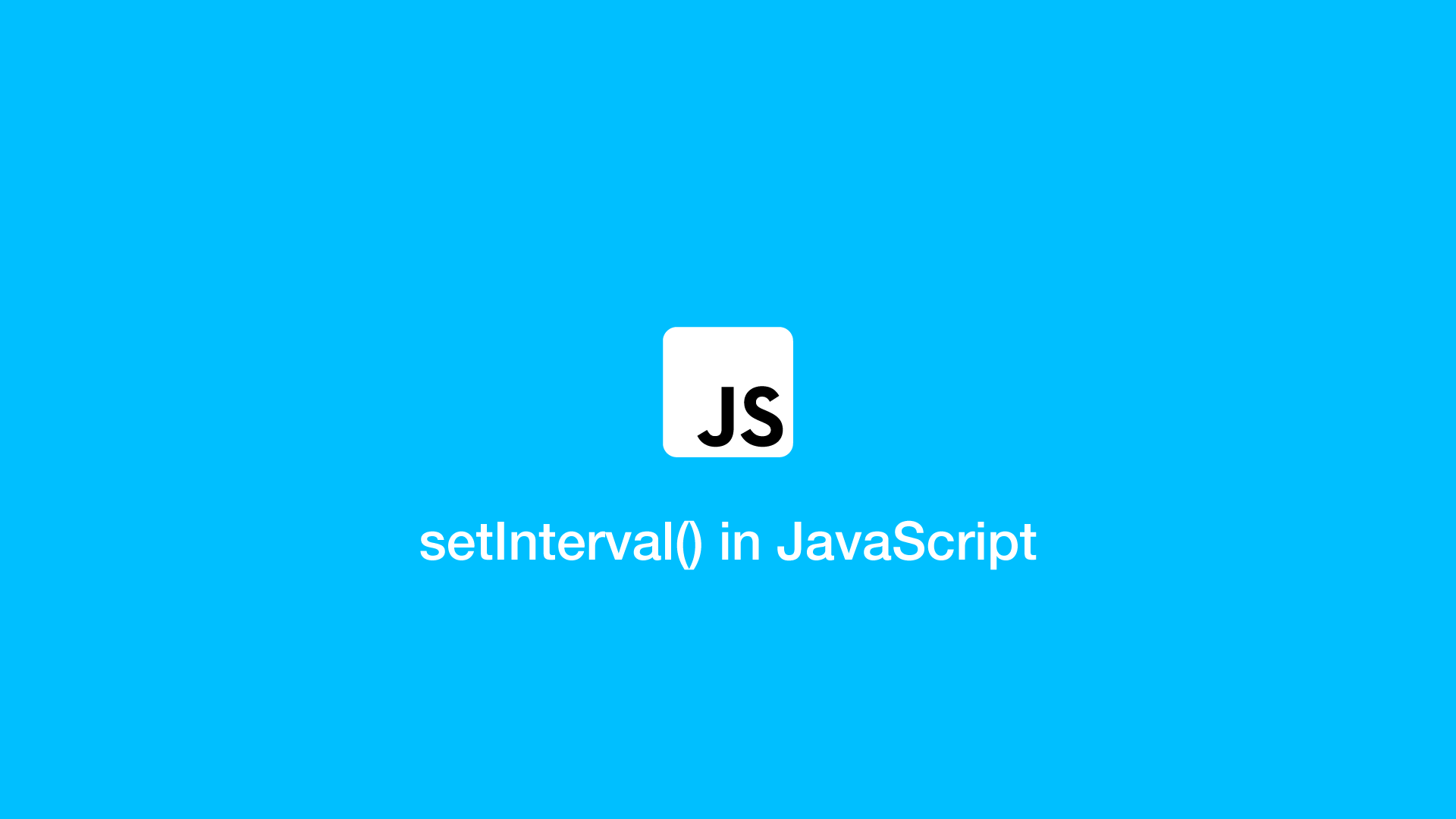# How to use setInterval() in JavaScriptThe `setInterval()` method in JavaScript repeats a function over an interval measured in milliseconds.

## setInterval() Syntax

The first argument of setInterval() is the function to run, followed by the millisecond interval. Optional arguments to pass to the function can be passed after the first two arguments.

``````setInterval(function, milliseconds, param1, param2)
``````

## setInterval() Example

Here is a basic example of a JavaScript `setInterval()` function that will run on page load and continue to repeat every one second indefinitely.

``````setInterval(function() {
console.log('hello');
}, 1000);
``````
``````hello
hello
...
``````

Note – 1000 milliseconds = 1 second.

## setInterval() with a Named Function

A common way to use `setInterval()` is with a named function, which allows the markup to be clearer and the interval to be instantiated easily from another location.

``````var myVar = setInterval(countSeconds, 1000);

function countSeconds() {

var d = new Date();
var s = d.getSeconds();

console.log(s);
}
``````
``````20
21
22
23
``````

## Pass Values back to setInterval()

Pass one or many values/variables to `setInterval()` after the interval duration argument.

``````var iteration = 0;

setInterval(function() {

console.log(iteration += 1);

}, 1000, iteration);
``````
``````1
2
3
``````

The same example as above but with a named function:

``````var iteration = 0;

var myVar = setInterval(myCount, 1000, iteration);

function myCount() {
console.log(iteration += 1);
}
``````

## How to stop setInterval() with clearInterval()

To stop/clear an interval, use the `clearInterval()` function and pass the variable name containing the interval object as the first argument.

To demonstrate this, let's create a function that will count to 5 at 1 second intervals then stop.

``````var iteration = 0;

var myVar = setInterval(myCount, 1000, iteration);

function myCount() {

iteration += 1

if (iteration == 5) {
clearInterval(myVar);
console.log('finished!');
}
}
``````
``````finished!
``````

#### Related Tutorials### How to Clear Interval in JavaScript

March 08, 2022### How to Create and Use Arrays in JavaScript

July 11, 2020### How to Read Files in JavaScript

October 16, 2020### How to Format a Date in JavaScript

August 27, 2020### How to Copy to Clipboard with JavaScript

September 07, 2020July 15, 2020